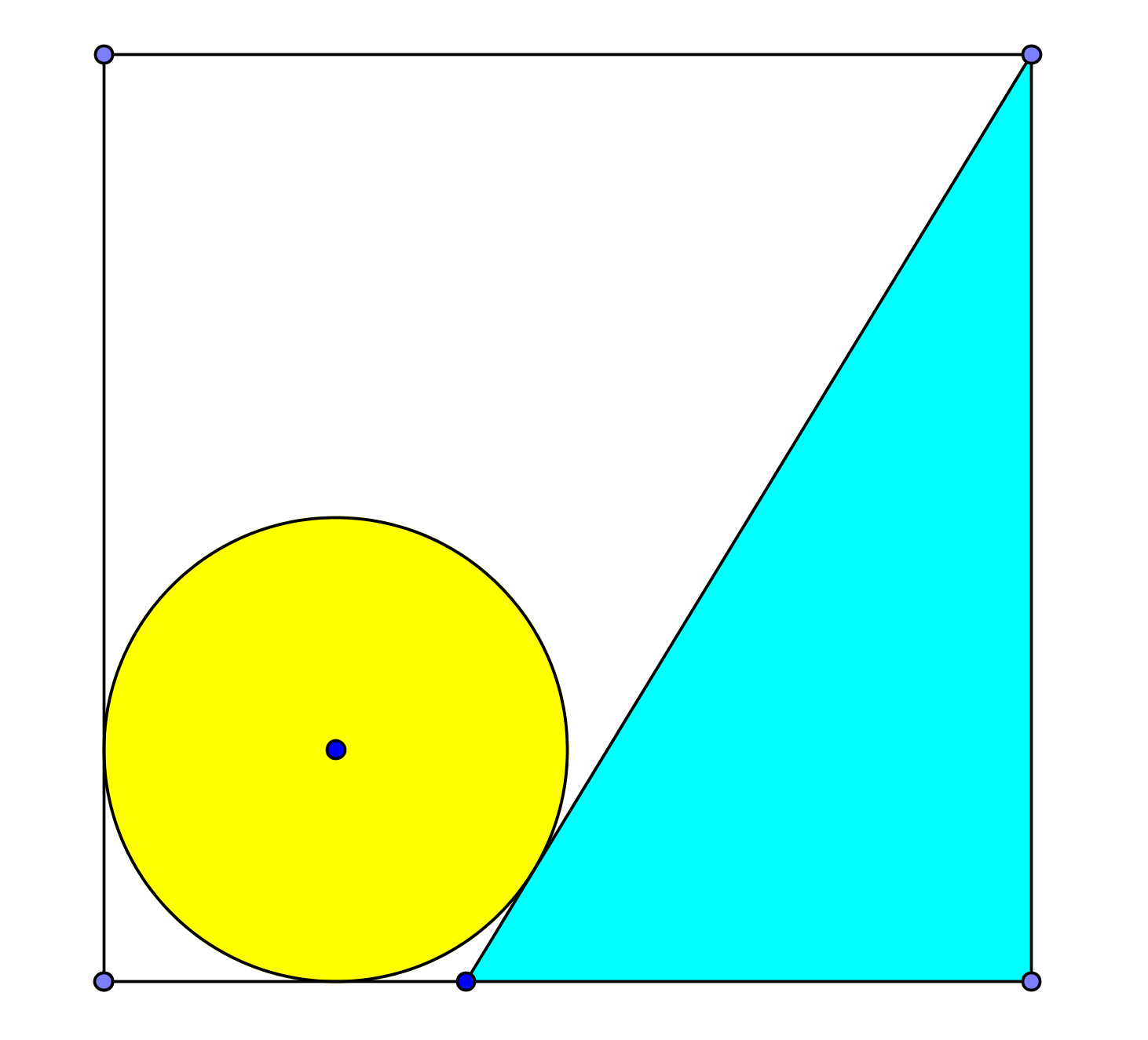# Sky Region

Geometry Level 5Find the area of the sky region if radius of circle is $\frac{1}{4}$ and each side of the square is 1.

The area of sky region is looks like as $\frac{a-\sqrt{b}}{c}$, where $a$, $b$, $c$ are positive integers and $b$ is square free then find $a+b-c$.

×